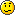# What is the reference value to calculate dBFS from an FFT of normalised audio?

DSP, Plug-in and Host development discussion.
KVRer
25 posts since 18 Dec, 2007
I am working on a spectral analyser and want to display the spectrum of an incoming signal. So far I have calculated the power using an FFT.
I then calculate the magnitude from the FFT output.

I know that to get the amplitude in dB the calculation is

amplitude = 20 * log10 ( mag )

to calculate the dBFS amplitude I use

amplitude = 20 * log10 ( mag / ref )

however I don't know what the reference value should be, since I am working with audio that has been normalised (range +1 to -1) and stored as a float.
Can anyone point me in the right direction?Thanks!

KVRist
212 posts since 1 Apr, 2009 from Hannover, Germany
There was a thread recently on this. I'll just repost my answer.A sine of Amplitude A will give you a bin magnitude of N * A / 2 (if sine frequency fits the bin exactly), where N is your FFT length. So if you want the dB scale in your analyzer to have some actual quantitative meaning, it would be appropriate to scale it so that a 0dB sine will give you a 0dB bin amplitude.

So your dB value would be 20 * log(2 * magnitude / N). That's what most analyzers do.

KVRer

Topic Starter

25 posts since 18 Dec, 2007
Brilliant. That's exactly what I neededThanks!